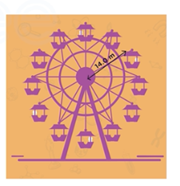Need Help?

Subscribe to Physics 1

###### \${selected_topic_name}
• Notes

$\begin{array}{l}{\text { The earth has a radius of } 6380 \mathrm{km} \text { and turns around once on its axis in } 24 \mathrm{h} \text { . (a) What is the radial }} \\ {\text { acceleration of an object at the earth's equator? Give your answer in } \mathrm{m} / \mathrm{s}^{\wedge}3 \text { and as a fraction of } \mathrm{g} \text { . }} \\ {\text { (b) If a-rad at the equator is greater than } \mathrm{g} \text { objects will fly off the earth's surface and into space. }} \\ {\text { What would the period of the earth's rotation have to be for this to occur? }}\end{array}$

$T=24 h$

$a_{r a d}=\frac{4 \pi^{2} R}{T^{2}}$

$a_{\text {rad }}=\frac{V^{2}}{R}=\frac{\left(2 \pi \frac{R}{7}\right)^{2}}{R}=\frac{4 \pi^{2} R}{T^{2}}$

$=\frac{4 \pi^{2}(6380 * 1000)}{\left((24 h)\left(3600 \frac{s}{h}\right)\right)^{2}}$

$=0.034 \mathrm{m} / \mathrm{s}^{2}$

$=3.4 * 10^{-3} g$

$a_{r a d}=g$

$T=\sqrt{\frac{4 \pi^{2} R}{a_{rad} }}=\sqrt{\frac{4 \pi^{2}(6380*1000)}{9.8}}$

$T=5070s=1.4 h$

$\begin{array}{l}{\text { A model of a helicopter rotor has four blaes, each } 3.40 \mathrm{m} \text { long from the central shaft to the blade tip. The }} \\ {\text { model is rotated in a wind tunnel at } 550 \text { rev/min. }} \\ {\text { (a) What is the linear speed of the blade tip, in } \mathrm{m} / \mathrm{s} \text { ? }} \\ {\text { (b) What is the radial acceleration of the blade tip expressed as a multiple of the acceleration of gravity, } \mathrm{g} ?}\end{array}$

$R=3.4 \mathrm{m}$

$a_{rad}=\frac{v^{2}}{R}$

$\text { 550 rev/min }=9.17 \mathrm{rev} / \mathrm{s} \quad \longrightarrow \quad \mathrm{T}=\frac{1}{9.17 \mathrm{rev} / \mathrm{s}}=0.109 \mathrm{s}$

(a) $v=\frac{2 \pi R}{T}=\frac{2 \pi(3.4)}{0.109}=196 \mathrm{m} / \mathrm{s}$

$a_{rad}=\frac{v^{2}}{R}=\frac{196^{2}}{3.4}=1.13 \times 10^{4}$

$=1.15 * 10^{3} g$

$\begin{array}{l}{\text { A Ferris wheel with radius } 14.0 \mathrm{m} \text { is turning about a horizontal axis through its center. The linear speed }} \\ {\text { of a passenger on the rim is constant and equal to } 7 \mathrm{m} / \mathrm{s} \text { . }} \\ {\text { What are the magnitude and direction of the passenger's acceleration as she passes through (a) the }} \\ {\text { lowest point in her circular motion? (b) The highest point in her circular motion? (c) How much time }} \\ {\text { does it take the Ferris wheel to make one revolution? }}\end{array}$$V \quad \text { constant }=7 \mathrm{m} / \mathrm{s}$

$R=14 \mathrm{m}$

${ a_{rad} } \ ??$

$T ? ?$

$V_{tan}=\frac{d|\vec{V}|}{d t}=0$

(a) $a_{r a d}=\frac{v^{2}}{R}=\frac{(7)^{2}}{14}=3.5 \mathrm{m/s}^{2}$ (upward)

(b) $a_{r ad} =? ? \quad=3.5 \mathrm{m/s}^{2}$

$T \ ?? \rightarrow T=\frac{2 \pi R}{V}$

$T=\frac{2 \pi(14)}{7}=12.6 \mathrm{s}$